Search by Topic

Resources tagged with Engineering similar to Diamonds Aren't Forever:

Filter by: Content type:
Age range:
Challenge level:

There are 59 results

Broad Topics > Applications > EngineeringThe Power of Dimensional Analysis

Age 14 to 18

An introduction to a useful tool to check the validity of an equation.Powerfully Fast

Age 16 to 18 Challenge Level:

Explore the power of aeroplanes, spaceships and horses.New Units for Old

Age 16 to 18 Challenge Level:

Can you match up the entries from this table of units?Go Spaceship Go

Age 16 to 18 Challenge Level:

Show that even a very powerful spaceship would eventually run out of overtaking powerStemnrich - Technology

Age 11 to 16 Challenge Level:

This is the technology section of stemNRICH - Core.Maths in the Undergraduate Physical Sciences

Age 16 to 18

An article about the kind of maths a first year undergraduate in physics, engineering and other physical sciences courses might encounter. The aim is to highlight the link between particular maths. . . .Battery Modelling

Age 16 to 18 Challenge Level:

Find out how to model a battery mathematically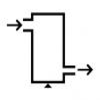Age 16 to 18 Challenge Level:

A look at a fluid mechanics technique called the Steady Flow Momentum Equation.Core Scientific Mathematics

Age 14 to 18 Challenge Level:

This is the area of the advanced stemNRICH site devoted to the core applied mathematics underlying the sciences.Ramping it Up

Age 16 to 18 Challenge Level:

Look at the calculus behind the simple act of a car going over a step.Moving Stonehenge

Age 16 to 18 Challenge Level:

A look at the fluid mechanics questions that are raised by the Stonehenge 'bluestones'.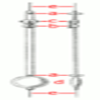The Not-so-simple Pendulum 2

Age 16 to 18 Challenge Level:

Things are roughened up and friction is now added to the approximate simple pendulumThe Not-so-simple Pendulum 1

Age 16 to 18 Challenge Level:

See how the motion of the simple pendulum is not-so-simple after all.Pumping the Power

Age 16 to 18 Challenge Level:

What is an AC voltage? How much power does an AC power source supply?Approximately Certain

Age 14 to 18 Challenge Level:

Estimate these curious quantities sufficiently accurately that you can rank them in order of sizeMach Attack

Age 16 to 18 Challenge Level:

Have you got the Mach knack? Discover the mathematics behind exceeding the sound barrier.Making More Tracks

Age 16 to 18 Challenge Level:

Given the equation for the path followed by the back wheel of a bike, can you solve to find the equation followed by the front wheel?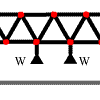More Bridge Building

Age 16 to 18 Challenge Level:

Which parts of these framework bridges are in tension and which parts are in compression?Model Solutions

Age 16 to 18 Challenge Level:

How do these modelling assumption affect the solutions?Dangerous Driver?

Age 16 to 18 Challenge Level:

Was it possible that this dangerous driving penalty was issued in error?Physnrich

Age 14 to 18 Challenge Level:

PhysNRICH is the area of the StemNRICH site devoted to the mathematics underlying the study of physicsEngnrich

Age 16 to 18 Challenge Level:

engNRICH is the area of the stemNRICH Advanced site devoted to the mathematics underlying the study of engineering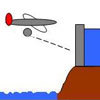Dam Busters 1

Age 16 to 18 Challenge Level:

At what positions and speeds can the bomb be dropped to destroy the dam?Random Squares

Age 16 to 18 Challenge Level:

What is a random pattern?Maximum Flow

Age 16 to 18 Challenge Level:

Given the graph of a supply network and the maximum capacity for flow in each section find the maximum flow across the network.Overarch 1

Age 16 to 18 Short Challenge Level:

This short question asks if you can work out the most precarious way to balance four tiles.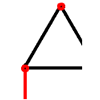Bridge Builder

Age 16 to 18 Challenge Level:

In this short problem we investigate the tensions and compressions in a framework made from springs and ropes.Dam Busters 2

Age 16 to 18 Challenge Level:

Can you work out which of the equations models a bouncing bomb? Will you be able to hit the target?AC/DC Circuits

Age 16 to 18

This article, including exercises, gives a thorough grounding in the topic of AC/DC circuits.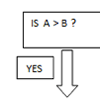Mystery Procedure

Age 14 to 16 Challenge Level:

Can you work out what this procedure is doing?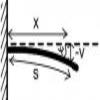Beam Me Up

Age 16 to 18 Challenge Level:

A preview of some of the beam deflection mechanics you will look at in the first year of an engineering degreeTurbo Turbines

Age 16 to 18 Challenge Level:

A look at power generation using wind turbines.Stonehenge Is Going Nowhere

Age 14 to 16 Challenge Level:

In an extension to the Stonehenge problem, consider the mechanical possibilities for an arrangement of frictional rollers.Impedance Can Be Complex!

Age 16 to 18 Challenge Level:

Put your complex numbers and calculus to the test with this impedance calculation.Differential Electricity

Age 16 to 18 Challenge Level:

As a capacitor discharges, its charge changes continuously. Find the differential equation governing this variation.The Wheatstone Bridge

Age 16 to 18 Challenge Level:

Explore the mathematics behind the famous Wheatstone Bridge circuit.Building up Friction

Age 16 to 18 Challenge Level:

A series of activities to build up intuition on the mathematics of friction.Not Another NAND!

Age 14 to 18 Challenge Level:

Prove that you can make any type of logic gate using just NAND gates.Euler's Buckling Formula

Age 16 to 18 Challenge Level:

Derive Euler's buckling formula from first principles.A Circuit Problem

Age 16 to 18 Short Challenge Level:

Explore the voltages and currents in this interesting circuit configuration.Stonehenge

Age 16 to 18 Challenge Level:

Explain why, when moving heavy objects on rollers, the object moves twice as fast as the rollers. Try a similar experiment yourself.Overarch 2

Age 16 to 18 Challenge Level:

Bricks are 20cm long and 10cm high. How high could an arch be built without mortar on a flat horizontal surface, to overhang by 1 metre? How big an overhang is it possible to make like this?Age 16 to 18 Challenge Level:

Can you set the logic gates so that this machine can decide how many bulbs have been switched on?Circular Circuitry

Age 16 to 18 Challenge Level:

What will happen when you switch on these circular circuits?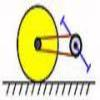Who Is an Engineer? A Quick Perspective After One Year of a University Engineering Course

Age 16 to 18

Doug has just finished the first year of his undergraduate engineering course at Cambridge University. Here he gives his perspectives on engineering.Procedure Solver

Age 16 to 18 Challenge Level:

Can you think like a computer and work out what this flow diagram does?